# 某屏蔽泵电机最大线圈温度热分析计算A Thermal Analysis for the Canned Motor Stator Winding Maximum Temperature

• 全文下载: PDF(751KB)    PP.254-260   DOI: 10.12677/MET.2019.83032
• 下载量: 237  浏览量: 350

In this document, there is the thermal analysis for the canned motor stator winding maximum temperature in nominal operating condition using the software MATLAB. First, the conservative analytical temperature value of annulus between the stator can and rotor can is obtained from the basic equation. Next, an axial thermal conduction model and many radial thermal conduction models for different axial locations are built, then the finite method is used for solving to get the sta-tor winding maximum temperature. After finishing the solution, the influence factors of maximum winding temperature are analyzed based on the model, and the influence factors on maximum winding temperature are obtained. After all, the analysis with using MATLAB software, of which calculation time is short, in order to ensure the accuracy of the basis, is a high-efficiency analysis.

1. 引言

2. 最大定子线圈温度计算

2.1. 分析目的

2.2. 分析方法

2.3. 计算模型说明

1) 由于定子铁心叠片的径向热传导能力远远大于轴向热传导能力，不考虑定子铁心叠片处的轴向热传导；

2) 由于定子线圈端部对机壳的空间距离不大，不考虑定子线圈端部对机壳的热辐射。考虑热辐射时，线圈端部的部分热量会以热辐射形式传导出去。因此，不考虑定子线圈端部的热辐射时计算的结果更为保守。

3) 电流在线圈中的涡流产生热量以等效系数进行修正。

2.3.1 .轴向传导模型

$k\frac{{\partial }^{2}T}{\partial {s}^{2}}+\stackrel{˙}{q}=0$ (1)

1) 电阻产生的热量可以通过线圈导体上的电流计算得到。单位长度线圈电阻产生的热流密度为：(导体截面积As)

${\stackrel{˙}{q}}_{1}=\rho \frac{{i}^{2}}{{A}_{s}{}^{2}}$ (2)

2) 根据牛顿冷却式，单位长度线圈的径向热传导方程：

${\stackrel{˙}{q}}_{2}=h\cdot {A}_{w}\cdot \left({T}_{w}-{T}_{\infty }\right)$ (3)

h：表面传热系数；

Aw：与流体接触的壁面面积；

Tw：线圈壁面的表面温度；

${T}_{\infty }$ ：流体温度。

$\frac{{\partial }^{2}T}{\partial {s}^{2}}=-\frac{1}{k}\left[\rho {\left(\frac{i}{{A}_{s}}\right)}^{2}-\frac{h{A}^{\prime }\left(T-{T}_{\infty }\right)}{{A}_{s}}\right]$ (4)

2.3.2. 径向传导模型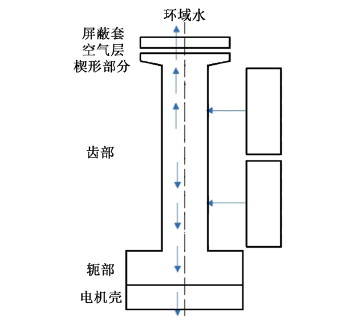Figure 1. Schematic for radial conductance calculation

$h=k/\delta$ (5)

$h{A}^{\prime }＝\frac{{\stackrel{˙}{q}}^{\prime }}{T-{T}_{\infty }}$ (6)

2.3.3. 定子屏蔽套表面强制对流换热系数

$\mathrm{Re}=\frac{2\delta v}{\nu }$ (7)

$\mathrm{Pr}=\frac{\nu }{\alpha }=\frac{\mu {c}_{p}}{k}$ (8)

$Nu=0.023{\mathrm{Re}}^{0.8}{P}_{r}{}^{0.3}$ (9)

$h=\frac{\lambda Nu}{2\delta }$ (10)

2.4. 线圈温度输入条件

1) 结构参数；

2) 损耗；

3) 各部件材料随温度变化的热传导系数；

4) 铜的电阻率(铜电阻率为0.0172 μΩ∙m)。

2.5. 温度边界条件

1) 电机壳外侧

2) 定转子环域水

$\Delta T=\frac{\stackrel{˙}{q}}{\stackrel{˙}{m}{c}_{p}}$Table 1. The temperature of the annulus

2.6. 最大线圈温度结果Table 2. The result of the thermal analysis, unit: ℃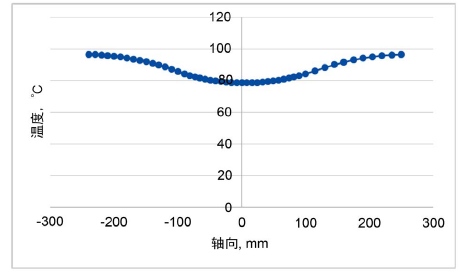Figure 2. Motor winding temperature distribution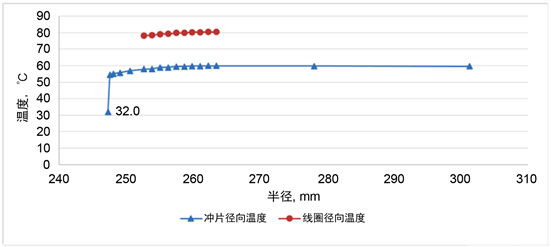Figure 3. The radial-distribution temperatureat the location of stator core

3. 最大线圈敏感性分析

1) 屏蔽套与冲片间的空间层厚度直接影响径向传导系数，对最大线圈温度影响较大，结果如图4所示。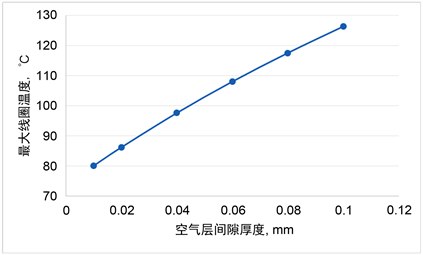Figure 4. The curved line between maximum winding temperature and air layer thickness

2) 屏蔽套表面的强制对流换热系数直接影响屏蔽套的温度，而对最大线圈温度影响几乎没有。最大线圈温度、屏蔽套温度与屏蔽套表面的强制对流换热系数关系曲线如图5所示。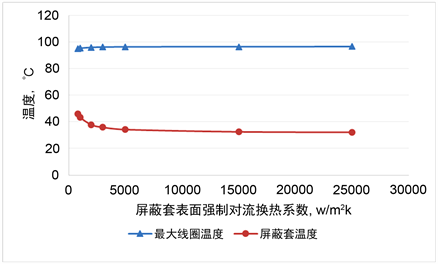Figure 5. The curvedline between the maximum winding temperature, can temperature and the forced convention heat transfer coefficient on inner surfaceof can

3) 小节：屏蔽套与冲片间的空气层厚度直接影响最大线圈的温度和其他各部件的温度；屏蔽套表面的强制热对流系数在一定范围内，仅会影响屏蔽套的温度，几乎对全局温度没有影响。

4. 总结

1) 由计算分析得到，最大线圈温度热分析模型可以忽略冲片的轴向热传导，忽略端部线圈的热辐射；

2) 该计算程序适用于铁芯槽部形状为狭窄等宽槽，对梨形槽计算精度不高；

3) 通过计算结果，得到屏蔽套与冲片间空气层厚度直接影响最大定子线圈温度；环域屏蔽套强制热对流系数，在一定范围内对最大线圈温度影响较小，对屏蔽套温度有一定的影响；

4) 该程序相对于有限元计算，计算速度快，无需建模，直接输入参数得出结果。

  霍菲, 张晓晨, 程鹏. 屏蔽电机定子温度场的数值计算与分析[J]. 电机与控制应用, 2006, 33(4): 7-12.  李伟力, 侯云鹏, 周封, 等. 大型水轮发电机定子股线导热的数值分析[J]. 中国电机工程学报, 2001, 21(4): 115-119.  于领新. 屏蔽电动机的计算特点[J]. 大电机技术, 1979(4): 24-30.  周建兴, 岂兴明, 矫津毅. MATLAB从入门到精通[M]. 北京: 人民邮电出版社, 2008.  杨世铭, 陶文铨. 传热学[M]. 北京: 高等教育出版社, 1998, 2006.